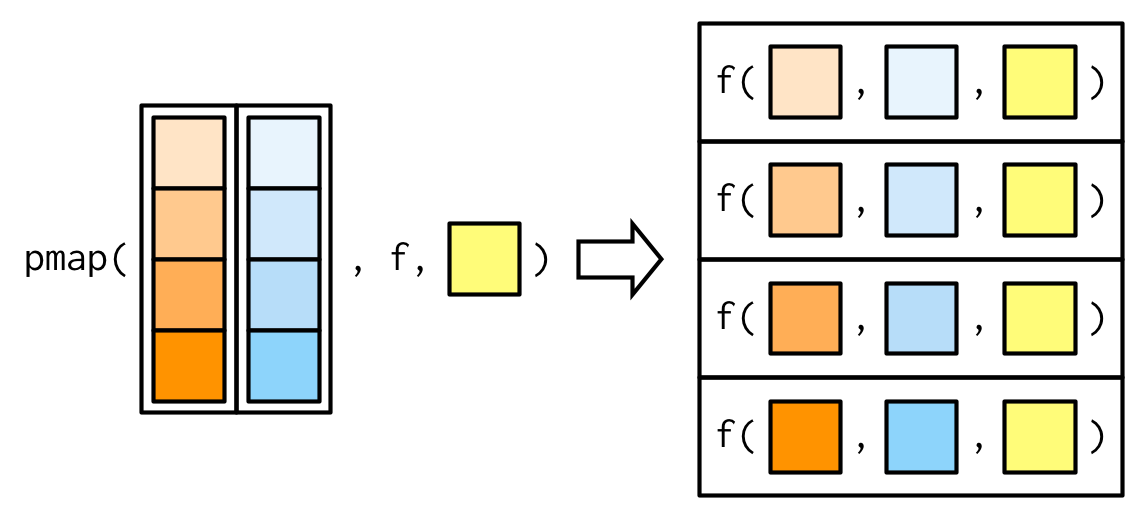# Looping over function arguments using purrr

``library(tidyverse)``

# Problem statement

Assume you have to call a function multiple times, but each with (possibly) different argument. Given enough repitioons, you will not want to repeat yourself.
In other words, we would like to loop over function arguments, each round in the loop giving the respective argument’value(s) to the function.

One example would be to generate many random values but each with different mean and/or sd:

``````rnorm(n = 1, mean = 0, sd = 1)
#>  -1.125083
rnorm(n = 2, mean = 0, sd = 2)
#>  2.542126 4.880299
rnorm(n = 3, mean = 0, sd = 3)
#>   0.3925394 -0.4260117  0.0236865
# heck, my wrists strains!``````

Note that some arguments may not change.

# Enter purrr

One way to build a loop is using `purrr`.

First we define the list of arguments.

``args <- list(sd = c(1, 2, 3), n = c(1, 2, 3))``

If we have some arguments that do not change, we can come up with a “prefilled” function, called a `partial` in purrr:

``rnorm_m0 <- partial(rnorm, mean = 0)``

With `pmap` we can map many lists (“values”, here: arguments and their values) to a function.

The following image taken from Hadley Wickham’s book Advanced R helps to visualize what `pmap` does:UPDATE

Mara Averick built a figure that illustrates the example of this post. Maybe more than words can, such a figure illustrate what `map()` and friends (here: `pmap())` do. Check out Mara’s cheatsheet on `purrr`, it’s super helpful.``````set.seed(42)
pmap(args, rnorm_m0)
#> []
#>  1.370958
#>
#> []
#>  -1.1293963  0.7262568
#>
#> []
#>   1.8985878  1.2128050 -0.3183735``````

Alternatively, we can directly hand-over the “constant” argument to `pmap`:

``````set.seed(42)
pmap(args, rnorm, mean = 0)
#> []
#>  1.370958
#>
#> []
#>  -1.1293963  0.7262568
#>
#> []
#>   1.8985878  1.2128050 -0.3183735``````